Next: conclusions Up: Continuity equations in the Previous: Constraints for the vector-isoscalar

## Constraints for the vector-isovector channel

Validity of the CE for the vector-isovector density, Eq. (30) for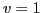and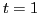, imposes through Eq. (48) constraints on the coupling constants that relate them in both spin and isospin spaces. At all the orders, we can express all dependent coupling constants through only one vector coupling constant, which can be chosen either in the set of the vector-isoscalar or vector-isovector ones. At second order, these constraints read,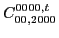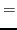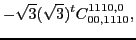(66)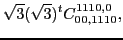(67)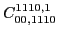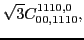(68)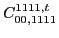(69)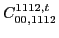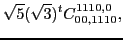(70)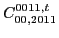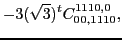(71)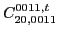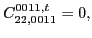(72)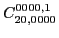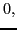(73)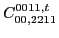(74)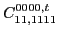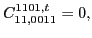(75)

and only coupling constant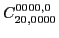is unrestricted. Altogether, at second order for the vector-isovector channel of the CE we have 2 free coupling constants and 11 coupling constants that are dependent. All the remaining 11 second-order coupling constants, which includes the surface ones in Eqs. (72)-(73), the tensor ones in Eq. (74) and spin-orbit ones in Eq. (75), are forced to be equal to zero.

For the fourth and sixth orders, analogous constraints are presented in Appendix C.Next: conclusions Up: Continuity equations in the Previous: Constraints for the vector-isoscalar
Jacek Dobaczewski 2011-11-11